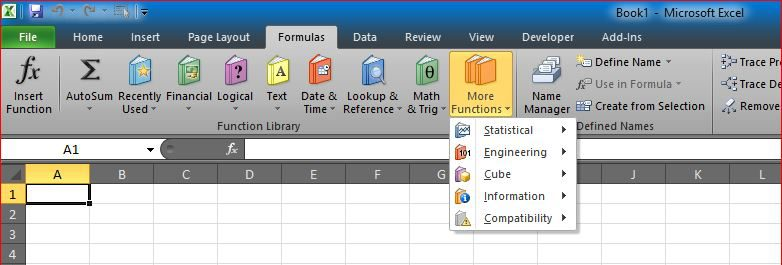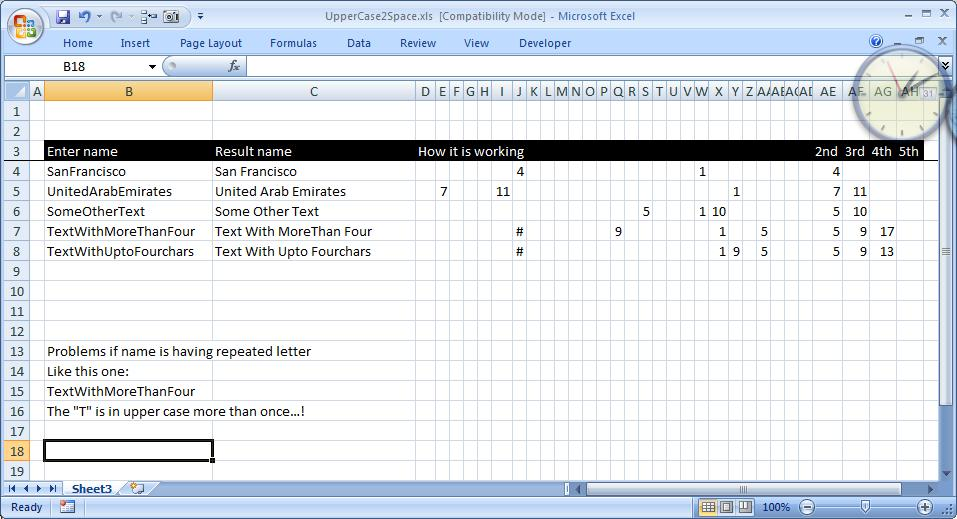# CamelCase to Camel CaseHere is a good practice for combining functions to get a full smartsheet

It basically add a space before any capital letter in a text, helpful when you have strings without spaces and want to convert them.

It is all in formulas. Put additional spaces before any upper case letter. made specially for custom request. Uses functions LEFT, MID, FIND, SMALL, COUNT, COLUMN, IF, CHAR, ISERROR, TRIM

I used here three formulas, first one in column C:

`=TRIM(LEFT(B4,AE4-1)&" "&MID(B4,AE4,IF(AF4="",500,AF4-AE4))&" "&IF(N(AF4)>0,MID(B4,AF4,IF(AG4="",500,AG4-AF4)),"")&" "&IF(N(AG4)>0,MID(B4,AG4,IF(AH4="",500,AH4-AG4)),""))`

And another one for columns E through AD:

`=IF(ISERROR(FIND(CHAR(COLUMN()+60),\$B4,1)),"",FIND(CHAR(COLUMN()+60),\$B4,1))`

And a third one in columns AE through AH

`=IF(COUNT(\$D4:\$AD4)>=COLUMN()-29,SMALL(\$D4:\$AD4,COLUMN()-29),"")`